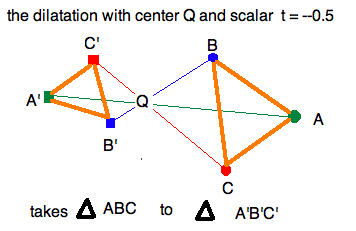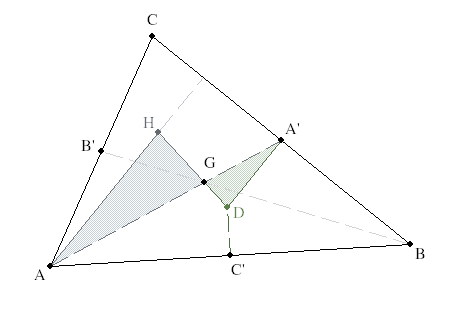Short Lesson on Dilatations
23sep11

\begin{document}
\maketitle\section{Introduction}
This lesson is a brief introduction into the concept of a \textit{dilatation}.
Note that this mathematical term has an extra "ta" in it. That emphasizes the
fact that we also allow "negative dilatations", as defined below.
The course
textbook devotes an entire chapter to this subject. Even the lesson in the
classnotes, which summarizes the chapter, is too long to fit into some
semester courses. Therefore, we present here an absolute minimum information
here to show how dilatations simplify the proof of Feuerbach's Theorem on
the \textit{ Nine Point Circle}.

This is possible because the effect of a dilatation on the plane can be
expressed with an explicit vector formula. This makes showing that dilatations
preserves barycentric coordinates easy. From this the chief properties of
a dilatations can be directly deduced.

\subsection{Two applications of Dilatation Theory}
First, we prove the Euler Line Theorem using a dilatations.
This complements Court's proof of the Euler Line Theorem using
Euclid's Elements, and permits us to compare the two styles of proof,
synthetic and analytic.

We finish
by proving \textit{Feuerbach's Theorem} using two dilatations. We will not
consider a synthetic proof of Feuerbach's Theorem, but there are plenty of
these on the WWW.

\section{Definition of Dilatations}
A dilatation is a transformation of the
plane to itself with takes every point $X$ another point $X'= Q+t(X-Q)$,
where $Q$ is the \textit{ center point} of the dilatation, and the real, but
nonzero number $t$ is the \textit{ scaling factor} of the dilatation.
When it is important to include the \textit{center} and \textit{scaling
factor} in the name of the dilatation, we use the functional notation
$\delta_{Q,t}(X)=Q+t(X-Q)$.

\subsection{Dilatations are bijective}
Note that a dilatation is a function taking the set of points in the plane
to itself. Since $t\ne0$, a dilatations is invertible because we can
solve the defining equation for $X = \frac{1}{t}X'+\frac{t-1}{t}Q$.
Hence this
function is bijective (one-to-one and onto). Functions of the plane
to itself that are bijection (i.e. one-to-one and ontor) are called
\textit{point transformations} of the plane.

\subsection{Geometrical interpretation}
Rewriting $X'=(1-t)Q + tX$ we see that a dilatation takes every point to
a different point on the line $\ell_{XQ}$. It also keeps its center point
fixed.

\subsection{Dilatations scale displacement vectors}
Solving $Y'-X' = (Q + t(Y-Q))-(Q + t(X-Q))= t(Y-X)$, we see that a
displacement vector is taken to a multiple of itself. From which
follows that:

\subsection{Dilatations preserve circles}
\textbf{Proof: }
Suppose we have take any point $R$ on the circumference of the circle
$\bigcirc(C,r)$. Applying the previous observation, all the
displacents $R-C$ (the radii) are transformed to $R'-C' = t(R-C)$,
which are the radii of $\bigcirc(C',|t|r)$. Since the outer ends of the
radii describe the circle, we have completed the proof.

\subsection{Dilatations preserve lines}
Given a dilatation $\delta_{Q,r}$, let $\ell_{AB}$ be any line.
For any point $X' \in \ell_{A'B'}$, calculate that

\begin{eqnarray*}
X' &=& aA' + bB' \\
&=&  a((1-t)Q + tA) + b((1-t)Q + tB) \\
&=&  (a+b)(1-t)Q  +t(aA +bB) \\
&=&  (1-t)Q + t(aA +bB) \\
&=&  \delta_{Q,t}(aA + bB) \\
&=&  \delta_{Q,t}(X) \\
\end{eqnarray*}

every point on the line $\ell_{A'B'}$  is the image under the dilatations of
a point on $\ell_{AB}$. This completes the proof. Note how
the condition $a+b=1$ figures in this deduction several times.
In other words, dilatation takes a line to a line which is parallel
to it.

\subsection{Dilatations preserve barycentric coordinates}

To show you have understood the basics above, you can work your way
through a proof of this fact here. The first step is to show that
if $\triangle ABC$ then $\triangle A'B'C'$, otherewise we could not
use the latter for a system of barycentric coordinates.

Question 1.
Which of the above properties lets you conclude that a dilatation
takes $\triangle ABC$ to a bona fide triangle $\triangle A'B'C'$
(and not, for instance,  to fewer than three distinct points, or
to three collinear points.)

This means we can measure the barycentric coordinates of a point relative
to two different triangels. How do we know they are the same? For dilatations
the coordiats do not change, although the positions do. That is because the
reference triangle is moved "the same way" as every other figure.

Question 2.
Now generalize the argument that dilatations take lines to parallel lines
to show that the barycentric
coordinates of $X'=\delta_{Q,t}(X)$ with respect to $\triangle A'B'C'$ are
identical with the barycentric coordinats of $X$ with respect to
$\triangle ABC$.

\section{Proof of Euler's Theorem}We start by interpreting the trisection of the medians in the Centroid
Theorem in terms of a dilatation centered at the barycenter $G$ and
negative scaling factor $-\frac{1}{2}$.

\begin{eqnarray*}
A' &=& \delta_{G,-\frac{1}{2}}(A)\\
B' &=& \delta_{G,-\frac{1}{2}}(B)\\
C' &=& \delta_{G,-\frac{1}{2}}(C)\\
\therefore \bigcirc A'B'C' &=& \delta_{G,-\frac{1}{2}}(\bigcirc ABC) \\
\end{eqnarray*}

This says that the circumcircle (the one through $A,B,C$) is in fact
the transform by  the dilatation of unique circle that passes through
the side bisectors $A',B',C'$. Can you deduce where it's center is?
But that is getting way ahead of the game!

Recall how we defined the orthocenter in terms of the circumcenter $D$,
from which we deduce the subsequent steps.
\begin{eqnarray*}
H &=& \delta_{G,-2}(D) \\
\therefore D &=& \delta_{G,-\frac{1}{2}}(H) \\
\therefore (DA') & \parallel & (AH) \\
\mbox{ and } (DB') & \parallel & (BH) \\
\mbox{ and } (DC') & \parallel & (CH) \\
\end{eqnarray*}

Since the LHS are perbises of the sides, the RHS are altitudes, and
we have the theorem without appealing to the simSAS theorem as we did
in Court's proof.

\section{Proof of Feuerbach's Theorem}
[To be continued here.]
\end{document}